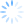# Brothers In Code

### ...a serious misallocation of .net resources

I needed to record the execution time in a stored proc.  I thought this would be straight forward until i realized the resolution on an oracle date datatype is only to the second.  Timestamp has the additional resolution (as well as timezone if  you want it).  Even cooler is that when you subtract two timestamps you get an interval datatype with the difference.  From there, however, I wanted to pass out the value in milliseconds, but that required each component of the interval to be extracted, multiplied, and added together.  Here's some sample code of the whole process.

``````declare  v_start timestamp := systimestamp;  v_end timestamp;  v_elapsed interval day to second(3);  v_elapsed_ms number;  i number;begin  for i in 1..100000 loop    v_elapsed_ms := 0;  end loop;
v_end := systimestamp;    v_elapsed := v_end - v_start;  v_elapsed_ms :=     ( extract(hour from v_elapsed)* 3600 +      extract(minute from v_elapsed)* 60 +      extract(second from v_elapsed) ) * 1000;
````    dbms_output.put_line('elapsed time:');  dbms_output.put_line(to_char(v_elapsed));  dbms_output.put_line(to_char(v_elapsed_ms));end;````